# The figure below shows a flexible rectangular raft foundation that is proposed for one of the...

The figure below shows a flexible rectangular raft foundation that is proposed for one of the industrial buildings at the site. The foundation is 10 feet X 20 feet and applies an uniform pressure of 3000 psf to the underlying soil.

a. Using the influence factor method to calculate the stress below a rectangular area, find the vertical stress increase (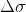) at 6 feet below points A, B, and C.

b. Assume that this foundation (thickness = 1.25 feet, Ef = 3.3 x 108 psf) is installed with the base of the foundation at a depth of 5 feet below the ground surface and the soil profile consists of 85 feet of sand (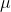s = 0.4, E0 = 4.1 x 105 psf, k = 10,500 lb/ft2/ft) overlying bedrock. Use the improved equation for elastic settlement in granular soils (Mayne and Poulos, 1999) to determine the elastic settlement of the sand layer under the center of the foundation.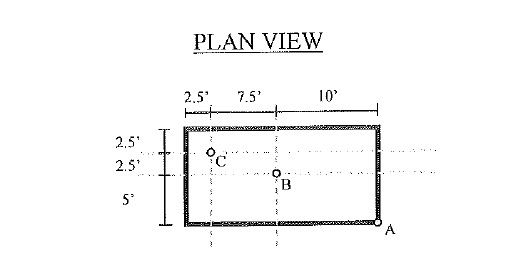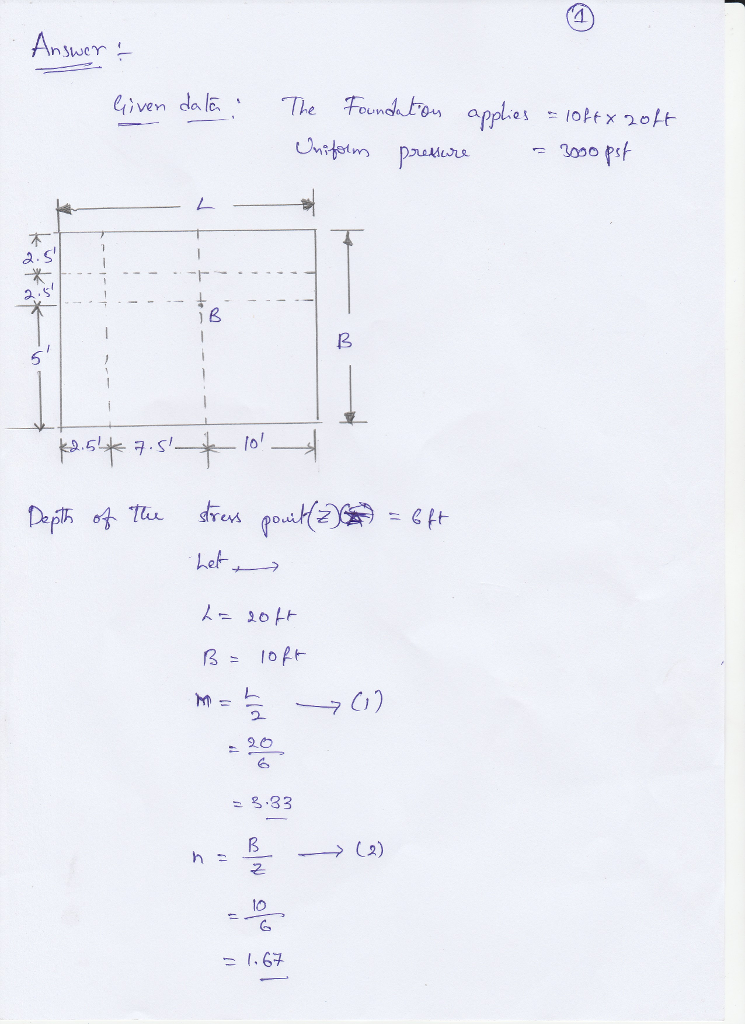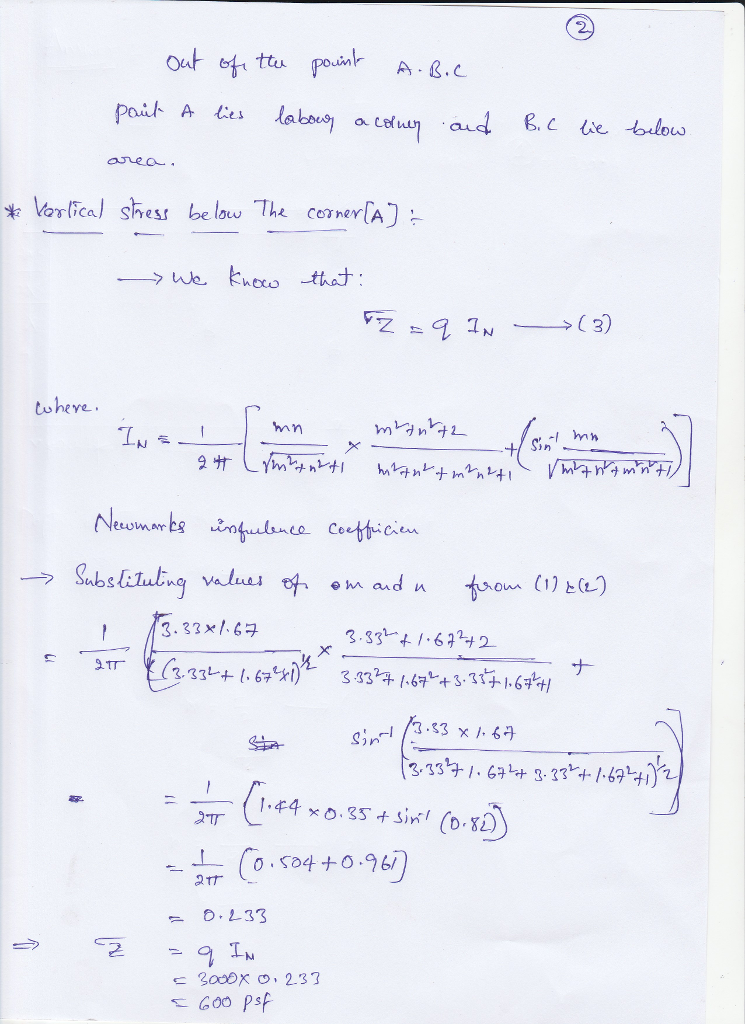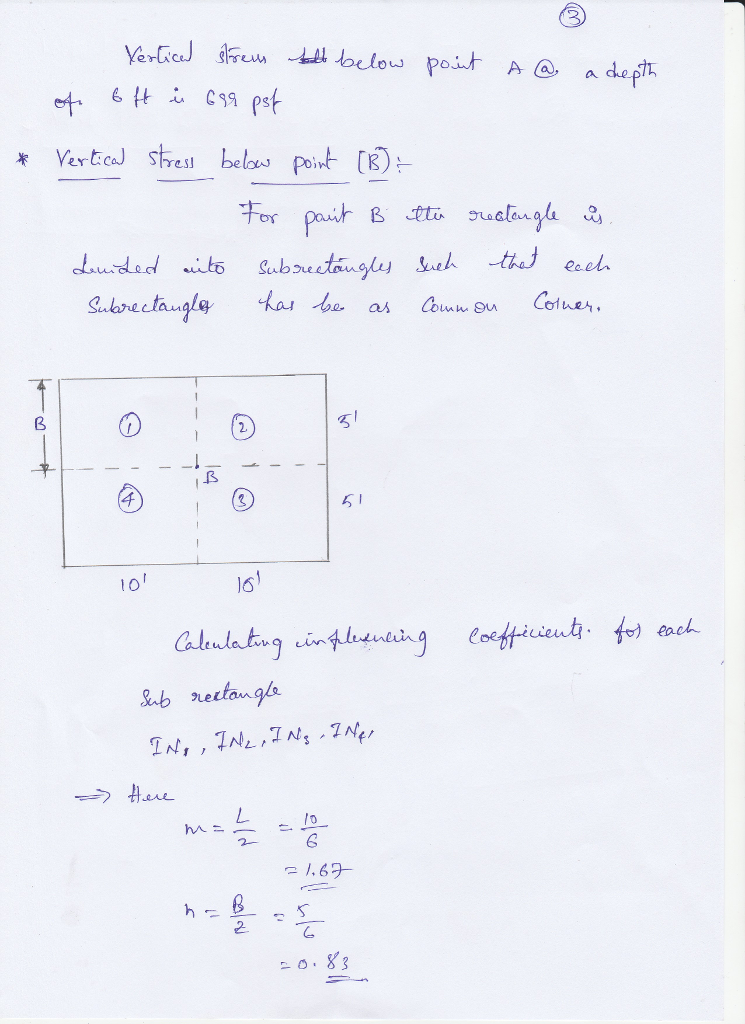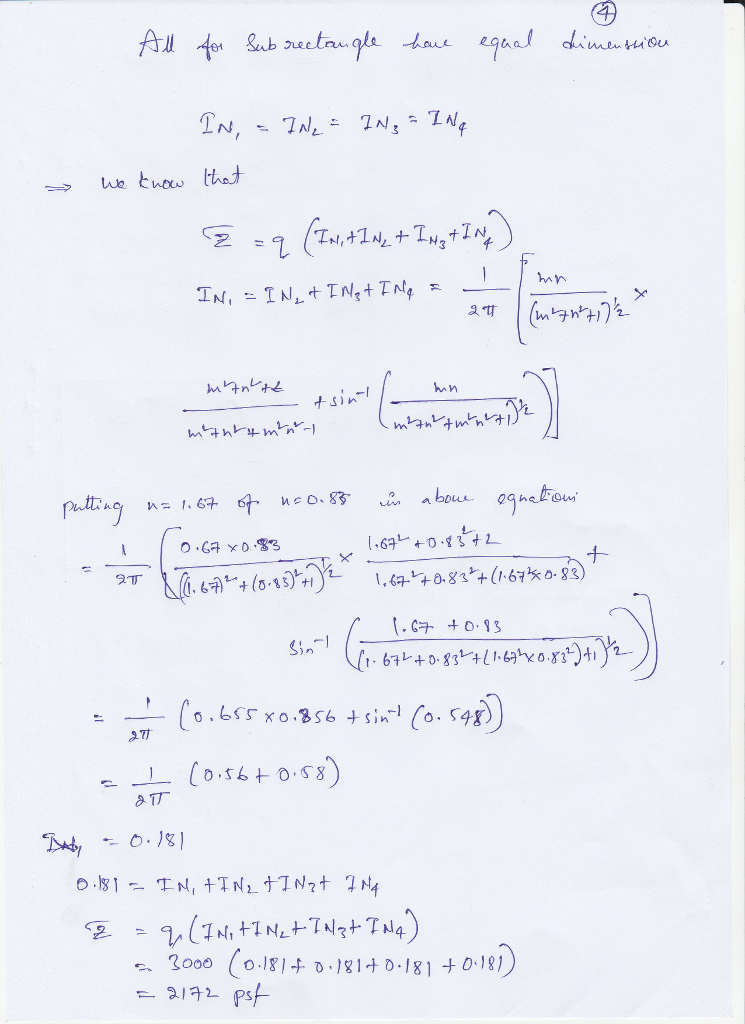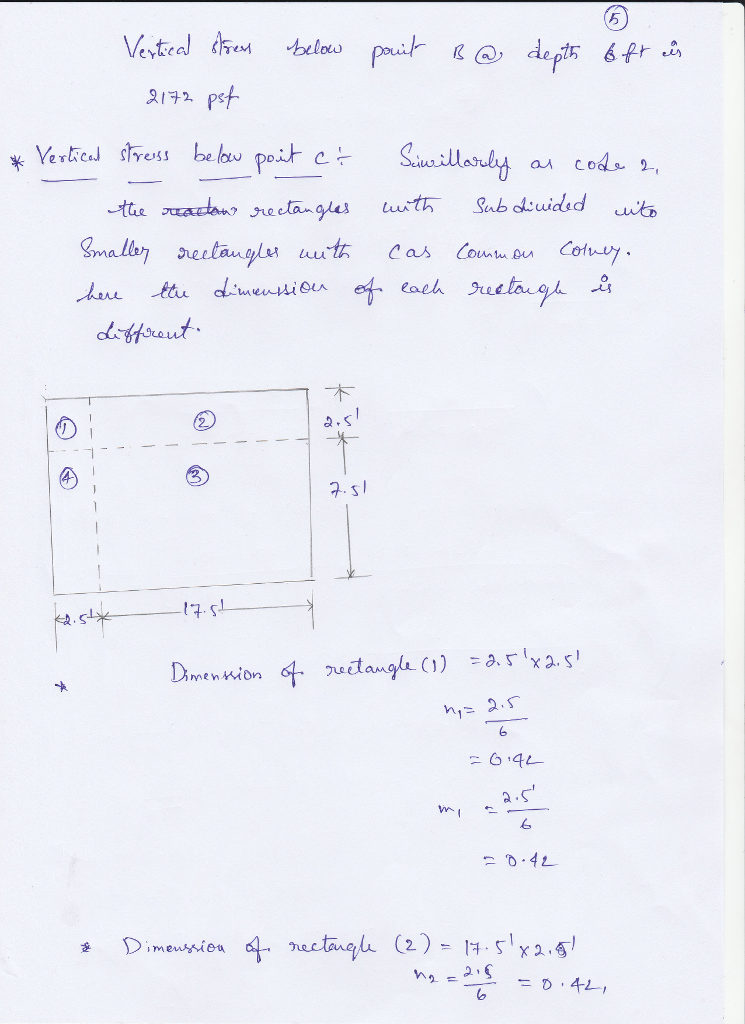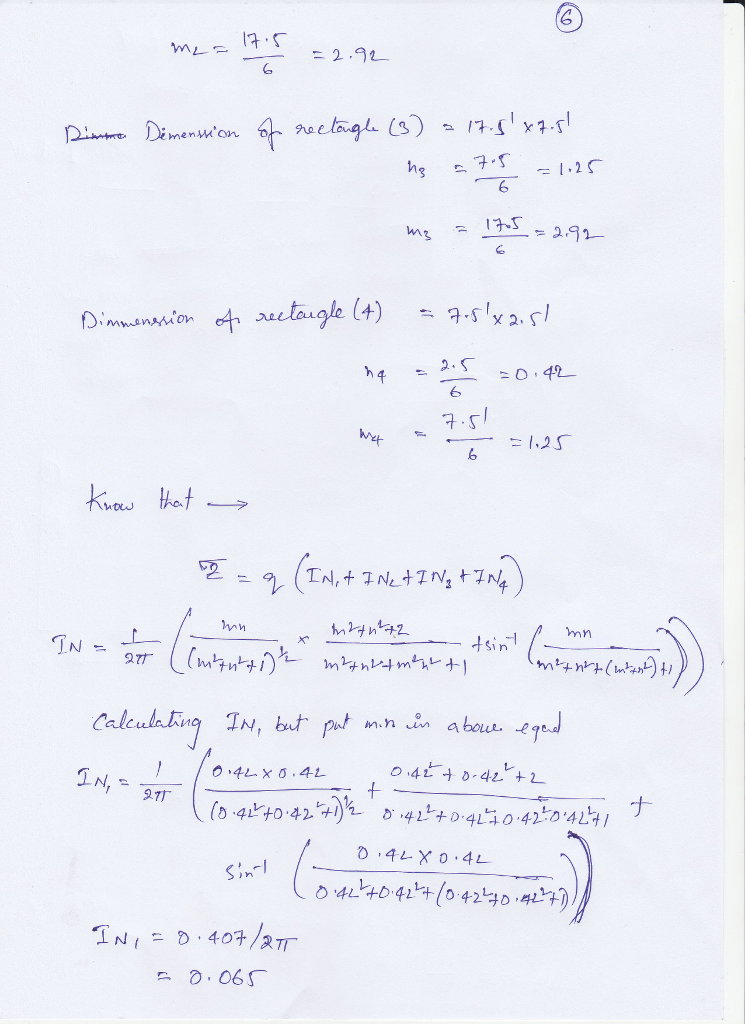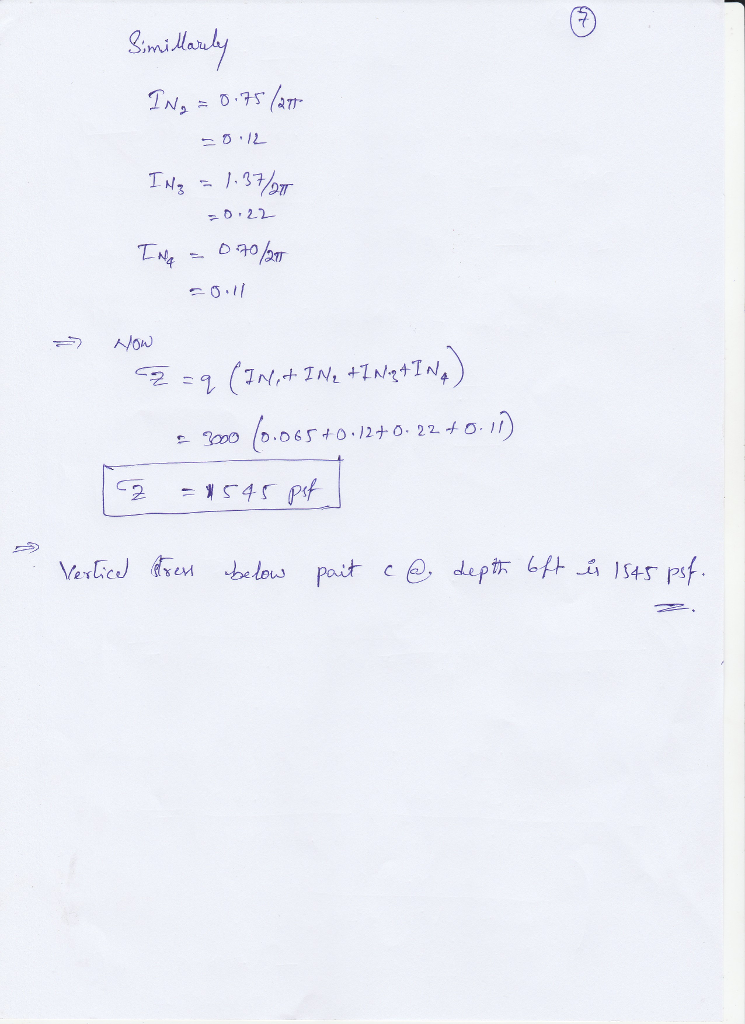#### Earn Coin

Coins can be redeemed for fabulous gifts.

Similar Homework Help Questions
• ### Figure P3 shows the soil profile at a site for a proposed office building. It is...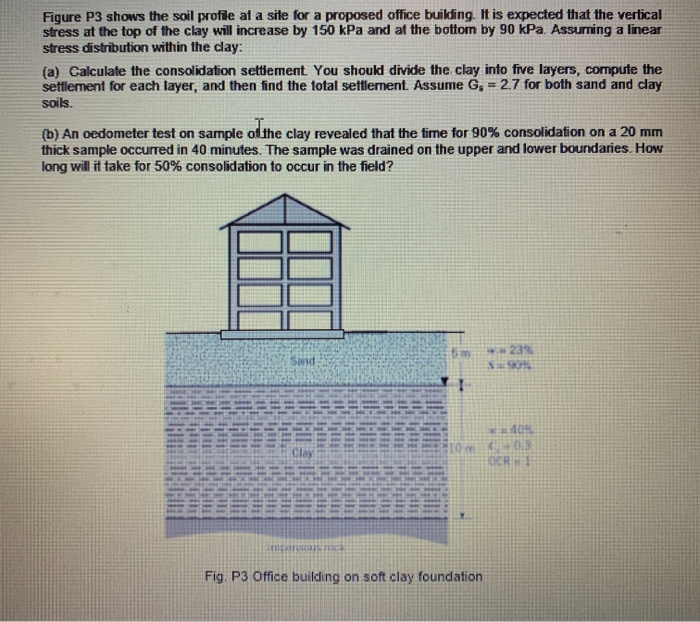Figure P3 shows the soil profile at a site for a proposed office building. It is expected that the vertical stress at the top of the clay will increase by 150 kPa and at the bottom by 90 kPa. Assurning a linear stress distribution within the clay: (a) Calculate the consolidation settlement. You should divide the clay into five layers, compute the settlement for each layer, and then find the total settlement. Assume G = 2.7 for both sand and...

• ### A high-rise structure is to be built over the soil profile shown below. The proposed rectangular...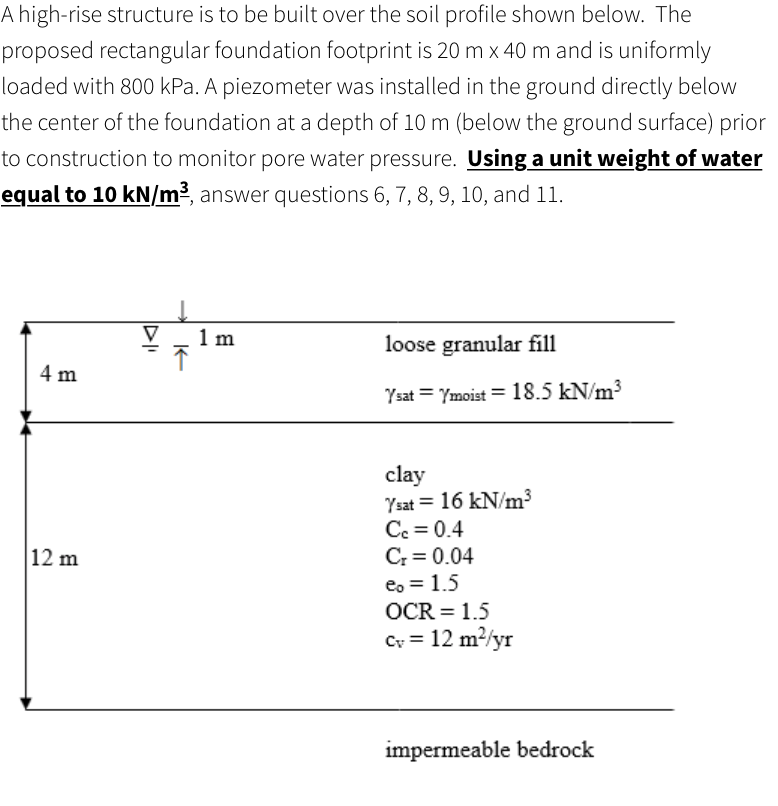A high-rise structure is to be built over the soil profile shown below. The proposed rectangular foundation footprint is 20 m x 40 m and is uniformly loaded with 800 kPa. A piezometer was installed in the ground directly below the center of the foundation at a depth of 10 m (below the ground surface) prior to construction to monitor pore water pressure. Using a unit weight of water equal to 10 kN/m], answer questions 6, 7, 8, 9, 10,...

• ### A 5m wide and 10m long rectangular foundation is built on a site where the soil...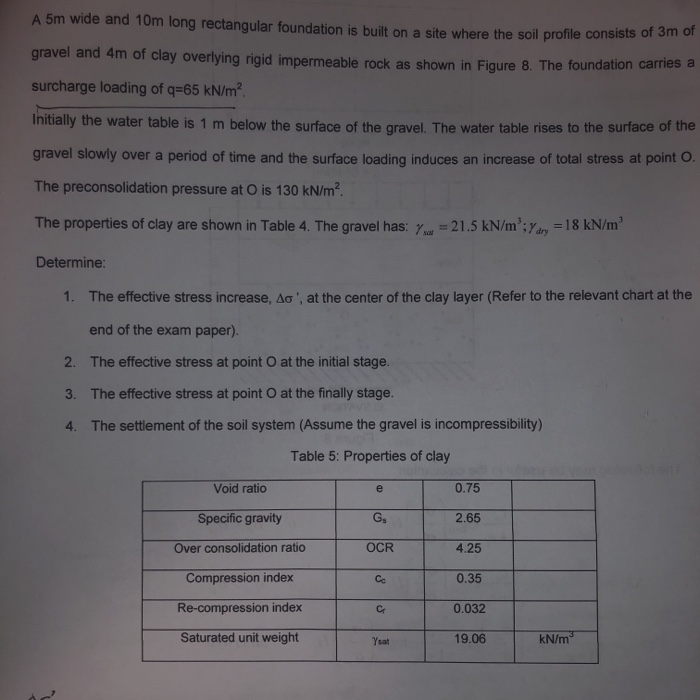A 5m wide and 10m long rectangular foundation is built on a site where the soil profile consists of 3m of gravel and 4m of clay overlying rigid impermeable rock as shown in Fiqure 8. The foundation carries a surcharge loading of q-65 kN/m2 Initially the water table is 1 m below the surface of the gravel. The water table rises to the surface of the gravel slowly over a period of time and the surface loading induces an increase...

• ### A 5m wide and 10m long rectangular foundation is built on a site where the soil...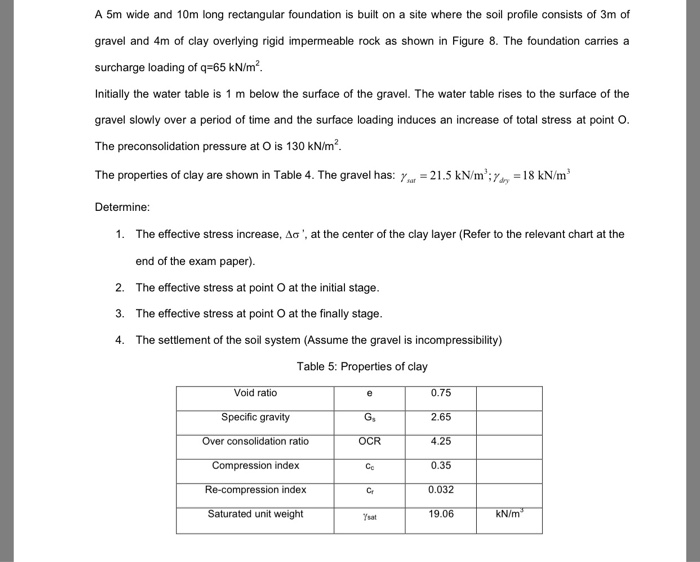A 5m wide and 10m long rectangular foundation is built on a site where the soil profile consists of 3m of gravel and 4m of clay overlying rigid impermeable rock as shown in Figure 8. The foundation carries a surcharge loading of q=65 kN/m2 Initially the water table is 1 m below the surface of the gravel. The water table rises to the surface of the gravel slowly over a period of time and the surface loading induces an increase...

Free Homework App